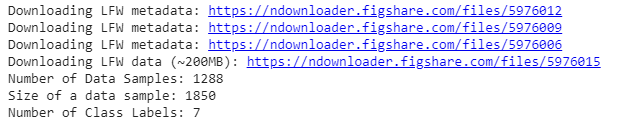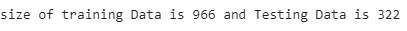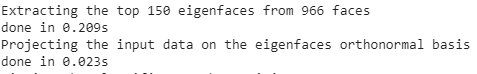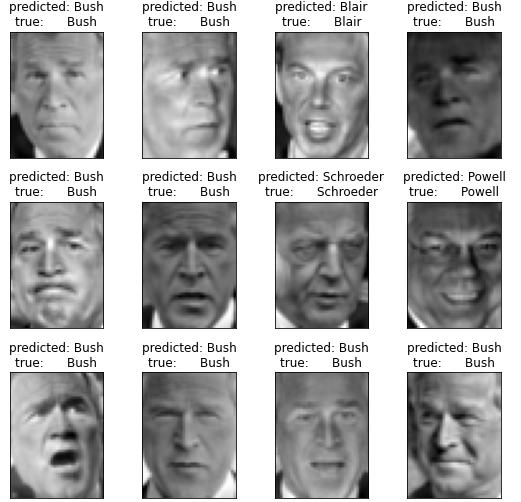# ML | Face Recognition Using PCA Implementation

Face Recognition is one of the most popular and controversial tasks of computer vision. One of the most important milestones is achieved using This approach was first developed by Sirovich and Kirby in 1987 and first used by Turk and Alex Pentland in face classification in 1991. It is easy to implement and thus used in many early face recognition applications. But it has some caveats such as this algorithm required cropped face images with proper light and pose for training. In this article, we will be discussing the implementation of this method in python and sklearn.
We need to first import the scikit-learn library for using the PCA function API that is provided into this library.
The scikit-learn library also provided an API to fetch LFW_peoples dataset. We also required matplotlib to plot faces.

Code: Importing libraries

 `# Import matplotlib library ` `import` `matplotlib.pyplot as plt ` ` `  `# Import scikit-learn library ` `from` `sklearn.model_selection ``import` `train_test_split ` `from` `sklearn.model_selection ``import` `GridSearchCV ` `from` `sklearn.datasets ``import` `fetch_lfw_people ` `from` `sklearn.metrics ``import` `classification_report ` `from` `sklearn.metrics ``import` `confusion_matrix ` `from` `sklearn.decomposition ``import` `PCA ` `from` `sklearn.svm ``import` `SVC ` ` `  `import` `numpy as np `

Now we import the LFW_people dataset using sklearn’s fetch_lfw_people function API. LFW_prople is the preprocess excerpt of LFW. It contains 13233 images of 5749 classes of shape 125 * 94. This function provides an parameter min_faces_per_person. This parameter allows us to select the classes that have at least min_faces_per_person different pictures. This function also has a parameter resize which resize every image in the extracted face. We use min_faces_per_person = 70 and resize = 0.4.

Code:

 `# this command will download the LFW_people's dataset to hard disk. ` `lfw_people ``=` `fetch_lfw_people(min_faces_per_person ``=` `70``, resize ``=` `0.4``) ` ` `  `# introspect the images arrays to find the shapes (for plotting) ` `n_samples, h, w ``=` `lfw_people.images.shape ` ` `  `# Instead of providing 2D data, X has data already in the form  of a vector that ` `# is required in this approach. ` `X ``=` `lfw_people.data ` `n_features ``=` `X.shape[``1``] ` ` `  `# the label to predict is the id of the person ` `y ``=` `lfw_people.target ` `target_names ``=` `lfw_people.target_names ` `n_classes ``=` `target_names.shape[``0``] ` ` `  `# Print Details about dataset ` `print``(``"Number of Data Samples: % d"` `%` `n_samples) ` `print``(``"Size of a data sample: % d"` `%` `n_features) ` `print``(``"Number of Class Labels: % d"` `%` `n_classes) `

Output:Code: Data Exploration

 `# Function to plot images in 3 * 4   ` `def` `plot_gallery(images, titles, h, w, n_row ``=` `3``, n_col ``=` `4``): ` `    ``plt.figure(figsize ``=``(``1.8` `*` `n_col, ``2.4` `*` `n_row)) ` `    ``plt.subplots_adjust(bottom ``=` `0``, left ``=``.``01``, right ``=``.``99``, top ``=``.``90``, hspace ``=``.``35``) ` `    ``for` `i ``in` `range``(n_row ``*` `n_col): ` `        ``plt.subplot(n_row, n_col, i ``+` `1``) ` `        ``plt.imshow(images[i].reshape((h, w)), cmap ``=` `plt.cm.gray) ` `        ``plt.title(titles[i], size ``=` `12``) ` `        ``plt.xticks(()) ` `        ``plt.yticks(()) ` ` `  `# Generate true labels above the images ` `def` `true_title(Y, target_names, i): ` `    ``true_name ``=` `target_names[Y[i]].rsplit(``' '``, ``1``)[``-``1``] ` `    ``return` `'true label:   % s'` `%` `(true_name) ` ` `  `true_titles ``=` `[true_title(y, target_names, i) ` `                     ``for` `i ``in` `range``(y.shape[``0``])] ` `plot_gallery(X, true_titles, h, w) `

Now, we apply train_test_split to split the data into training and testing sets. We use 25% of the data for testing.
Code: Splitting the dataset

 `X_train, X_test, y_train, y_test ``=` `train_test_split( ` `    ``X, y, test_size ``=` `0.25``, random_state ``=` `42``) ` `print``(``"size of training Data is % d and Testing Data is % d"` `%``( ` `        ``y_train.shape[``0``], y_test.shape[``0``])) `Now, we apply the PCA algorithm on the training dataset which computes EigenFaces. Here, we take n_components = 150 means we extract the top 150 Eigenfaces from the algorithm. We also print the time taken to apply this algorithm.
Code: Implementing PCA

 `n_components ``=` `150` ` `  `t0 ``=` `time() ` `pca ``=` `PCA(n_components ``=` `n_components, svd_solver ``=``'randomized'``, ` `          ``whiten ``=` `True``).fit(X_train) ` `print``(``"done in % 0.3fs"` `%` `(time() ``-` `t0)) ` ` `  `eigenfaces ``=` `pca.components_.reshape((n_components, h, w)) ` ` `  `print``(``"Projecting the input data on the eigenfaces orthonormal basis"``) ` `t0 ``=` `time() ` `X_train_pca ``=` `pca.transform(X_train) ` `X_test_pca ``=` `pca.transform(X_test) ` `print``(``"done in % 0.3fs"` `%` `(time() ``-` `t0)) `The above code generates the EigenFace and each image is represented by a vector of size 1 * 150. The values in this vector represent the coefficient corresponding to that Eigenface. These coefficient are generated using transform function on the function.

Eigenfaces generated by this PCA algorithm:

Code: Explore the coefficient generated by the above algorithm.

 `print``(``"Sample Data point after applying PCA\n"``, X_train_pca[``0``]) ` `print``(``"-----------------------------------------------------"``) ` `print``(``"Dimesnsions of training set = % s and Test Set = % s"``%``( ` `        ``X_train.shape, X_test.shape)) `

```Sample Data point after applying PCA
[-2.0756025  -1.0457923   2.126936    0.03682641 -0.7575693  -0.51736575
0.8555038   1.0519465   0.45772424  0.01348036 -0.03962574  0.63872665
0.4816719   2.337867    1.7784412   0.13310494 -2.271292   -4.4569106
2.0977738  -1.1379385   0.1884598  -0.33499134  1.1254574  -0.32403082
0.14094219  1.0769527   0.7588098  -0.09976506  3.1199582   0.8837879
-0.893391    1.1595601   1.430711    1.685587    1.3434631  -1.2590996
-0.639135   -2.336333   -0.01364169 -1.463893   -0.46878636 -1.0548446
-1.3329269   1.1364135   2.2223723  -1.801526   -0.3064784  -1.0281631
4.7735424   3.4598463   1.9261417  -1.3513585  -0.2590924   2.010101
-1.056406    0.36097565  1.1712595   0.75685936  0.90112156  0.59933555
-0.46541685  2.0979452   1.3457304   1.9343662   5.068155   -0.70603204
0.6064072  -0.89698195 -0.21625179 -2.1058862  -1.6839983  -0.19965973
-1.7508434  -3.0504303   2.051207    0.39461815  0.12691127  1.2121526
-0.79466134 -1.3895757  -2.0269105  -2.791953    1.4810398   0.1946961
0.26118103 -0.1208623   1.1642501   0.80152154  1.2733462   0.09606536
-0.98096275  0.31221238  1.0365396   0.8510516   0.5742255  -0.49945745
-1.3462409  -1.036648   -0.4910289   1.0547347   1.2205439  -1.3073852
-1.1884091   1.8626214   0.6881952   1.8356183  -1.6419449   0.57973146
1.3768481  -1.8154184   2.0562973  -0.14337398  1.3765801  -1.4830858
-0.0109648   2.245713    1.6913172   0.73172116  1.0212364  -0.09626482
0.38742945 -1.8325268   0.8476424  -0.33258602 -0.96296996  0.57641584
-1.1661777  -0.4716097   0.5479076   0.16398667  0.2818301  -0.83848953
-1.1516216  -1.0798892  -0.58455086 -0.40767965 -0.67279476 -0.9364346
0.62396616  0.9837545   0.1692572   0.90677387 -0.12059807  0.6222619
-0.32074842 -1.5255395   1.3164424   0.42598936  1.2535237   0.11011053]
-----------------------------------------------------
Dimesnsions of training set (966, 1850) and Test Set (322, 1850)
```

Now we use Support Vector Machine (SVM) as our classification algorithm. We train the data using the PCA coefficient generated in previous steps.

Code: Applying Grid Search Algorithm

 `print``(``"Fitting the classifier to the training set"``) ` `t0 ``=` `time() ` `param_grid ``=` `{``'C'``: [``1e3``, ``5e3``, ``1e4``, ``5e4``, ``1e5``], ` `              ``'gamma'``: [``0.0001``, ``0.0005``, ``0.001``, ``0.005``, ``0.01``, ``0.1``], } ` `clf ``=` `GridSearchCV( ` `    ``SVC(kernel ``=``'rbf'``, class_weight ``=``'balanced'``), param_grid ` `) ` `clf ``=` `clf.fit(X_train_pca, y_train) ` `print``(``"done in % 0.3fs"` `%` `(time() ``-` `t0)) ` `print``(``"Best estimator found by grid search:"``) ` `print``(clf.best_estimator_) ` ` `  `print``(``"Predicting people's names on the test set"``) ` `t0 ``=` `time() ` `y_pred ``=` `clf.predict(X_test_pca) ` `print``(``"done in % 0.3fs"` `%` `(time() ``-` `t0)) ` `# print classifiction results ` `print``(classification_report(y_test, y_pred, target_names ``=` `target_names)) ` `# print confusion matrix ` `print``(``"Confusion Matrix is:"``) ` `print``(confusion_matrix(y_test, y_pred, labels ``=` `range``(n_classes))) `

```Fitting the classifier to the training set
done in 45.872s
Best estimator found by grid search:
SVC(C=1000.0, break_ties=False, cache_size=200, class_weight='balanced',
coef0=0.0, decision_function_shape='ovr', degree=3, gamma=0.005,
kernel='rbf', max_iter=-1, probability=False, random_state=None,
shrinking=True, tol=0.001, verbose=False)
Predicting people's names on the test set
done in 0.076s
precision    recall  f1-score   support

Ariel Sharon       0.75      0.46      0.57        13
Colin Powell       0.79      0.87      0.83        60
Donald Rumsfeld       0.89      0.63      0.74        27
George W Bush       0.84      0.98      0.90       146
Gerhard Schroeder       0.95      0.80      0.87        25
Hugo Chavez       1.00      0.47      0.64        15
Tony Blair       0.97      0.81      0.88        36

accuracy                           0.85       322
macro avg       0.88      0.72      0.77       322
weighted avg       0.86      0.85      0.84       322

Confusion Matrix is :
[[  6   3   0   4   0   0   0]
[  1  52   1   6   0   0   0]
[  1   2  17   7   0   0   0]
[  0   3   0 143   0   0   0]
[  0   1   0   3  20   0   1]
[  0   3   0   4   1   7   0]
[  0   2   1   4   0   0  29]]

```

So, our accuracy is 0.85 and Results of our prediction are:Reference:

My Personal Notes arrow_drop_upCheck out this Author's contributed articles.

If you like GeeksforGeeks and would like to contribute, you can also write an article using contribute.geeksforgeeks.org or mail your article to contribute@geeksforgeeks.org. See your article appearing on the GeeksforGeeks main page and help other Geeks.

Please Improve this article if you find anything incorrect by clicking on the "Improve Article" button below.

Article Tags :
Practice Tags :

Be the First to upvote.

Please write to us at contribute@geeksforgeeks.org to report any issue with the above content.